Non-Parametric Estimation of a Single Inflection Point in Noisy Observed Signal | OMICS International
Journal of Electrical & Electronic Systems

# Non-Parametric Estimation of a Single Inflection Point in Noisy Observed Signal

Nezamoddin N. Kachouie1* and Armin Schwartzman2

1Department of Mathematical Sciences, Florida Institute of Technology, USA

2Department of Statistics North Carolina State University Raleigh, NC

*Corresponding Author:
Nezamoddin N. Kachouie
Department of Mathematical Sciences
Florida Institute of Technology, USA
E-mail: [email protected]

Received Date: May 28, 2013; Accepted Date: July 01, 2013; Published Date: July 03, 2013

Citation: Kachouie NN, Schwartzman A (2013) Non-Parametric Estimation of a Single Inflection Point in Noisy Observed Signal. J Electr Electron Syst 2:108. doi:10.4172/2332-0796.1000108

Copyright: © 2013 Kachouie NN, et al. This is an open-access article distributed under the terms of the Creative Commons Attribution License, which permits unrestricted use, distribution, and reproduction in any medium, provided the original author and source are credited.

Visit for more related articles at Journal of Electrical & Electronic Systems

#### Abstract

Inflection point detection is an important yet challenging problem in science and engineering. This paper addresses the estimation of a single inflection point location in noisy observations using non-parametric polynomial regression. To address the bandwidth problem, a constrained approach is proposed to ensure having a single inflection point, thereby reducing the uncertainty in the inflection point location whereas being flexible on the shape of the underlying signal. The performance of the proposed method is evaluated through simulations. It is shown that the proposed method can effectively estimate the inflection point under high noise conditions.

#### Keywords

Inflection point estimation; Local polynomial regression; Bandwidth selection; Cross validation

#### Introduction

Detection of abrupt changes, including inflection points, in the observed noisy signals is an important and challenging problem in engineering and science. Several methods have been proposed to solve this problem and proved to be successful for specific applications. Yet, there is demand for new and general approaches that are applicable to broader range of applications. The change point detection problem can be divided into two categories; i) sequential or on-line, and ii) batch or off-line. In on-line methods, the observed data are inspected sequentially to catch a change as soon as it occurs. These methods are mainly used in quality control, real time surveillance & vision systems, and real time fault detection in computer networks [1-6]. In offline methods the entire data set is observed and can be processed at once. The off-line problems are growing fast and have attracted many researchers in computational biology and biostatistics as well as offline computer vision applications [7-14]. In previous work [15,16], often Cross Validation (CV) is used to select the bandwidth to locate the discontinuities in the observed signal including inflection points, change points, jumps and valleys. Discontinuities in , assuming an unknown number, are located by detecting the zero crossing of the second derivative and the local maxima of the first derivative while CV is used to estimate bandwidth parameters. Bootstrap is used in  for designing a nested CV method for detecting the change points by finding the local maxima of the first derivative while considering the number of change points is unknown.

For smooth underlying signals, a change point may be defined as an inflection point, i.e. a point where the second derivative of the signal changes sign. In this work, we focus on the problem of estimating the location of an inflection point when it is known that only one such point exists in the underlying signal. When considering smoothing methods for estimating the underlying signal, standard methods for bandwidth selection, such as cross validation, are designed to optimally estimate the underlying function, and can thus produce many inflection points; creating uncertainty in the location of the true inflection point. Our goal is to design a fast and simple, yet effective method to address the inflection point detection. The interest here is to detect a single significant inflection point in either entire range or a fragment of observed signal. A non-parametric inflection point detection method is proposed in which we smooth the noisy observations and locate the inflection point using the estimated zero crossing of the second derivative of the smoothed curve. Spatial curve fitting in our case is accomplished by applying local polynomial regression to smooth the noisy data. We propose a constrained method for bandwidth selection intended to allow a single inflection point, thereby it increases the accuracy of the estimated inflection point location, whereas it permits fitting a flexible function and avoids over-smoothing. Using non-parametric regression allows estimating the standard error of the located inflection point to evaluate the accuracy of the estimation. The performance of the method is evaluated through simulations using a sigmoid function that is corrupted by adding different levels of Gaussian noise.

The proposed method is discussed in the next section by describing local polynomial regression, inflection point detection, and optimal bandwidth selection. The results are then demonstrated, compared with cross validation are discussed at the end.

#### Methods

Nonparametric polynomial regression

Suppose that n pairs of observations

(s,Yi),,i = 1,2,…,n (1)

consist of a response variable and a location and are related by the signal plus noise model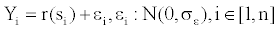(2)

where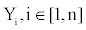are observed noisy samples, εi is Gaussian noise ,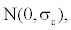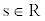, and r is an unknown underlying regression function. The regression function r can be locally approximated at the point s by a polynomial of order p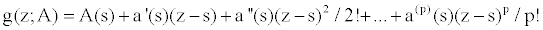(3)

where z is a point in a neighborhood of s. We use least squares to estimate the polynomial coefficient vector .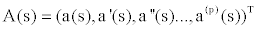The estimated polynomial coefficient vector is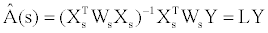(4)

where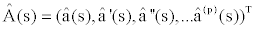is obtained by minimization of the least square problem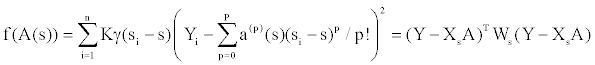(5)

where si is a point in a neighborhood of s,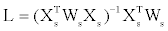is the smoothing matrix, Xs is a n×(P +1) matrix.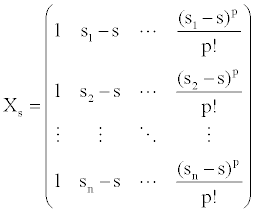(6)

and Wsis an n× n diagonal matrix.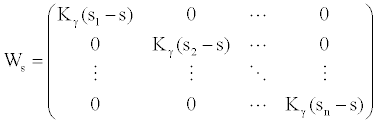(7)

and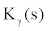is a kernel with bandwidth γ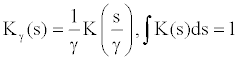(8)

To estimate r(s)=a(s), the inner product of the first row of with is computed by L with Y is computed by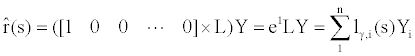(9)

where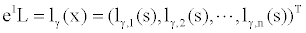. The variance of this estimator for independent noise that is of interest here is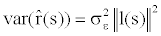(10)

Similarly, the derivatives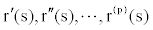can be estimated by the inner product of Y with the second row, third row, and (P+1)th row of L respectively.

Inflection point detection

An inflection point is a point (D) on the estimated curve where the curvature changes sign. Here we discuss the positive inflection point where the curvature changes from being concave upward to being concave downward, i.e.,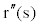crosses the zero line from being positive (> 0) to being negative (< 0) and the first derivative has a local maximum (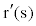> 0) there. In a similar way to (9), the estimated 2nd derivative (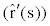) is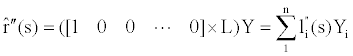(11)

and its associated standard error can be computed by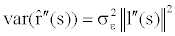(12)

The negative inflection point can be identified in a similar way where the second derivative changes sign from negative to positive. As illustrated in Fig. 1, the standard error of the estimated inflection point location is approximated by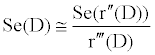(13)

where D is the identified inflection point location,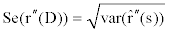is the standard error of the estimated second derivative at the inflection point based on (12), and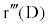is the estimated third derivative at the inflection point, computed in a similar way as (9) and (11).

Optimal bandwidth selection

Conventionally, the optimal bandwidth is chosen using the leaveone- out cross validation score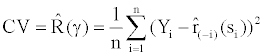(14)

where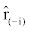is estimated by excluding ith pair (si, Yi). The optimal bandwidth chosen by cross validation may produce many inflection points. As an illustration, a sigmoid function is corrupted with different noise levels and is estimated by small, large (Figure 1), and optimal bandwidth chosen by cross validation (Figure 2). Applying the selected optimal bandwidth obtained by cross validation, we may identify zero, one, or several inflection points (Figure 3 and 4) and it is difficult to identify the main one as the estimation of the true inflection point of the original unknown curve (sigmoid function here).

Figure 1: Sigmoid function corrupted with different noise levels: no noise (first row), low noise (second row), and high noise (third row). True sigmoid (blue), noisy observations (purple dots), and estimated sigmoid (black) applying a small bandwidth (left) and applying a large bandwidth (right).

Figure 2: (Left column) Cross validation curve for different noise levels: no noise (first row), low noise (second row), and high noise (third row). (Right column) True sigmoid (blue), noisy observations (purple dots), and estimated sigmoid applying the optimal bandwidth chosen by cross validation (black).

Figure 3: Located inflection point for different levels of independent Gaussian noise: no noise (first row), low noise (second row), and high noise (third row). (Left column) Zero down-crossings of the second derivative of the estimated sigmoid (red) vs. the zero line (yellow). (Right column) True sigmoid (blue), noisy observations (purple dots), and estimated sigmoid (black) applying the optimal bandwidth chosen by cross validation with the located inflection points superimposed as yellow disks.

Figure 4: (Top row) Cross validation curve (left) and the estimated sigmoid applying the optimal bandwidth chosen by cross validation (right) for a typical realization. (Middle row) Located inflection point for 500 simulations applying the optimal bandwidth chosen by cross validation (left) and the number of detected inflection points for each simulation (right). (Bottom row) The distribution of the locations of the inflection points for 500 simulations (left) and the distribution of the number of inflection points for the same 500 simulations.

The new proposed method applies a constraint for bandwidth selection to ensure that the smoothed curve (by the selected bandwidth) has only one inflection point. The bandwidth γ is the smallest bandwidth producing the smoothed curve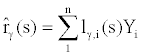(15)

that satisfies the constraint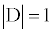(16)

where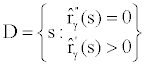(17)

is the set of zero down-crossings of the second derivative. Equation 17 ensures that the number of positive inflection points of the smoothed curve applying bandwidth γ is one.

#### Results and Discussion

The proposed method is applied to a simulated sigmoid function which is corrupted by adding different levels of independent Gaussian noise. For comparison, first we show the application of the regression-based inflection point detection where the bandwidth is selected by cross validation. The results obtained by applying the proposed constrained bandwidth selection are then presented. Finally the performance is quantified by running several hundreds of simulation instances.

Bandwidth selection by cross validation

A sigmoid function was simulated with s = [0,6000]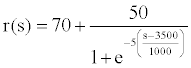(18)

and corrupted with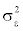= 0 (no noise),= 1.85 (low noise with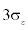= 81.6% of the sigmoid height), and= 185 (high noise with= 81.6% of the sigmoid height). The estimated smooth curves for small (γ = 200) and large (γ =1000) bandwidths for different noise levels are depicted in Figure 1. The cross validation curve and the smoothed curve applying the selected bandwidth identified by minimum cross validation score for different noise levels is shown in Figure 2. The optimal bandwidth (γopt) is 100 for no noise, 150 for low noise and 400 for high noise. The number of detected inflection points is 1, 8, and 2 for different noise levels respectively (Figure 3). Although the inflection point closest to the true one is among the detected inflection points, further processing is needed to identify it. The identification of the main inflection point is even more challenging and prone to error when the detected candidate inflection points have close values of their estimated first derivative (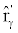).

Next, the sigmoid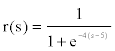was simulated and independent Gaussian noise with= 0.01 (medium noise with 3σ = 30% of the sigmoid height) was added. Five hundred independent simulation instances were performed, inflection points were located, and the number of inflection points was counted for each simulation. The cross validation curve and the smoothed curve applying the optimal cross validation bandwidth for a typical simulation (γopt) is shown in Figure 4 (First row). The location of inflection points and the number of detected inflection points for the five hundred runs are depicted in Figure 4 (Middle row). The distribution (histogram) of inflection point location and the number of detected inflection points are shown in Figure 4 (Bottom row). Applying the optimal cross validation bandwidth, up to seven inflection points was identified (Figure 4).

Bandwidth selection by the proposed method

To avoid detecting multiple false inflection points, the proposed method was applied to select the optimal bandwidth while ensuring to have only one inflection point in the smoothed curve. Independent Gaussian noise with different levels= 0 (no noise),= 1.85 (low noise),= 185 (high noise), and= 1665 (very high noise) was added to corrupt the signal. The noisy sigmoid was then estimated using the non-parametric regression where the bandwidth was selected by the proposed method (Figure 5). Regardless of the noise level, the significant inflection point was closely estimated (Figure 5 (Right column)).

Figure 5: Located inflection point by the proposed method for different levels of independent Gaussian noise: first row (no noise), second row (low noise), third row (high noise), and fourth row (very high noise). (Left column) zero down-crossings of the second derivative of the estimated sigmoid (red) vs. the zero line (yellow). (Right column) True sigmoid (blue), noisy observations (purple dots), and estimated sigmoid (black) using the optimal constrained bandwidth where the located inflection points are superimposed as yellow disks.

Figure 6 further illustrates the inflection point detection and the estimation error under high noise conditions. The confidence interval was computed by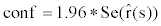and is superimposed in red where the standard error (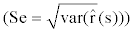) of the smoothed curve was computed using (10). For high level of Gaussian noise with= 2 272.25 or equivalently= 50 that is equal to 100% of sigmoid height (Figure 6 (Top)), the detected inflection point is located at s = 3441.4 which is close to the true inflection point at s = 3550 with 1.67% estimation error. Even at very high noise conditions with= 2500 so that= 150 is equal to 300% of sigmoid height (Figure 6 (Bottom)), the detected inflection point is located at s = 3395 which is only 3% away from the true inflection point location.

Figure 6: True sigmoid (blue), noisy observations (purple), estimated sigmoid applying the proposed method (black), confidence interval (red), and the true (yellow disk) and the detected inflection point (red disk) where the true sigmoid function is corrupted with Gaussian noise. (Top) High noise with 3 50 100% ε σ = = of sigmoid height. (Bottom) Very high noise with 3 150 300% ε σ = = of sigmoid height.

Performance of the proposed method

The performance of the proposed method is assessed by comparing the location of the identified inflection point vs. the true inflection point location (D = 5; r(D) = 0.5) through several hundred simulations where the sigmoid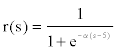was corrupted with high level of independent Gaussian noise. The simulations were repeated for six different sigmoid slopes (α). Two hundred simulations were performed for each slope, starting with more challenging case of fairly shallow slope α = 0.5 to sharp slope α = 3.

The detected inflection point, standard error, true mean squared error, and the coverage of estimated confidence interval for the detected inflection points were estimated for different slopes (Figure 7). The mean of the detected inflection points closely follows the true inflection point location (D = 5) for different slopes (Figure 7 (Top)). As it can be observed, while the slope increases from low on the left to high on the right, estimation precision increases (Figure 7 (Middle)). In addition, the estimated standard errors (box plots) closely match the true mean square error computed from the 200 simulations. The 95% coverage depicted by the black disks (Figure 7 (Bottom)) was computed for each slope by the percentage of the number of simulation instances for which the true inflection point

Figure 7: (Top) Estimated location of the inflection points for six different slopes with 200 simulations instances per slope. (Middle) Estimated log squared standard error associated with the detected inflection point locations and log true mean squared error (green disks) for the same simulation instances. (Bottom) The coverage of confidence interval of estimated inflection point locations for the same simulation instances.

(D = 5) satisfies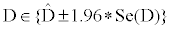(19)

The point-wise confidence interval of the coverage was then estimated (red disks) for each slope using the Binomial distribution applying the number of simulation instances (Figure 7 (Bottom)).

Insights

The proposed method selects the optimal bandwidth to ensure a single inflection point. This avoids the uncertainty due to detection of zero or multiple inflection points when applying standard bandwidth selection methods such as cross validation. Therefore the proposed method increases the accuracy of the estimated inflection point location for such applications where the true function has only one inflection point. This method permits a flexible fit to estimate the underlying function by taking advantage of non-parametric regression while avoiding over-smoothing.

Figure 8 illustrates the basic mechanism under a low noise with= 25 or equivalently= 15 that is equal to 30% of sigmoid height. Starting from a small bandwidth, the proposed method gradually increases the bandwidth to smooth out false inflection points. The bandwidth increases from left to right: left = 100, middle = 300, and right = 600. Shallow inflection points disappear by applying larger bandwidths. We should point out that there is a wide range of large bandwidths for which there is only one inflection point. We propose selecting the smallest bandwidth within the range that guarantees only one inflection point in order to reduce over-smoothing caused by applying the larger bandwidths.

Figure 8: Searching for the smallest bandwidth to satisfy (16). Sigmoid is corrupted by adding Gaussian noise and the estimated curve is shown for different bandwidths, starting from small (100) and increasing the bandwidth to meet (16). True sigmoid (blue), noisy observations (purple), estimated function (black), the detected inflection points (red disks) and the true inflection point (yellow disk). (Top row) Estimated sigmoid and detected inflection points. (Middle row) First derivative of the estimated function. (Bottom row) Second derivative of the estimated function.

Note from Figure 6 that the confidence bands do not necessarily cover the true function. In other words, the estimation of the function is biased. However, this is not a concern in our case because our interest is in estimating the inflection point location, not the underlying function. The gain in accuracy in estimating the inflection point location is worth the extra bias in the estimation of the function.

The estimation of the inflection point location and its standard error, however, are nearly unbiased. This can be observed in Figure 7 (Top) and Figure 7 (Middle), which show that the estimated inflection point locations are close in average to the true value, and that the estimated log squared standard errors are close in average to the true log mean square errors. Figure 7 (Bottom) further shows for all slopes larger than α = 1.5 that the coverage of the 95% confidence interval for the inflection point location is indeed at least the nominal (95%). For shallow slopes (α = 0.5 & 1), the region in which the true function transitions from low to high is larger, making the detection of the inflection point harder. In this case, the estimation of the inflection point is still unbiased but the coverage of the confidence intervals drops below 90% for such challenging cases.

#### References

Select your language of interest to view the total content in your interested language

### Article Usage

• Total views: 13033
• [From(publication date):
December-2013 - Jan 18, 2020]
• Breakdown by view type
• HTML page views : 9221Can't read the image? click here to refresh